# 2OA3 Video1

##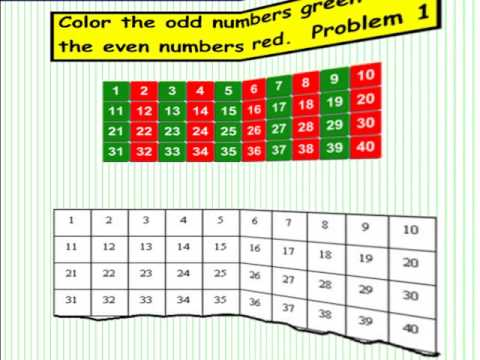By Mrhoffywon02

2.OA.3Determine whether a group of objects (up to 20) has an odd or even number of members, e.g., by pairing objects or counting them by 2s; write an equation to express an even number as a sum of two equal addends# Division - Sharing Equally: Grade 3

##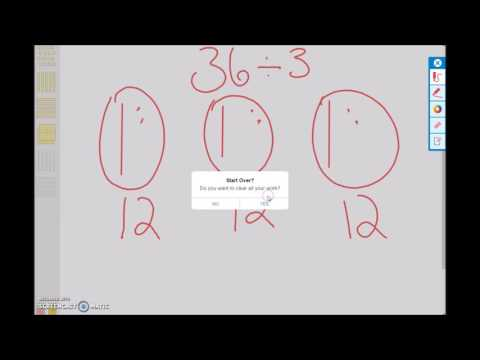By university of Houston mathematics education

Use multiplication and division within 100 to solve word problems in situations involving equal groups, arrays, and measurement quantities, e.g., by using drawings and equations with a symbol for the unknown number to represent the problem.# Division - Sharing Equally: Grade 3

##By university of Houston mathematics education

Use multiplication and division within 100 to solve word problems in situations involving equal groups, arrays, and measurement quantities, e.g., by using drawings and equations with a symbol for the unknown number to represent the problem.# Division Problem Solving

##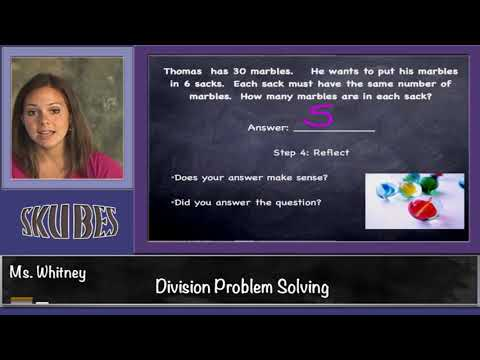By Skubes ed

Use multiplication and division within 100 to solve word problems in situations involving equal groups, arrays, and measurement quantities, e.g., by using drawings and equations with a symbol for the unknown number to represent the problem.1# [3.OA.2-1.0] Basic Division - Common Core Standard - Word Problem

##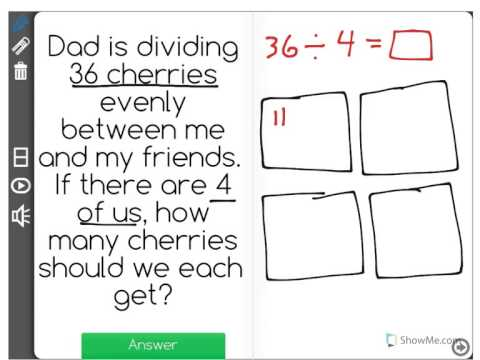By Front Row

Discover more Common Core Math at https://www.frontrowed.comInterpret whole-number quotients of whole numbers, e.g., interpret 56 ÃƒÂ· 8 as the number of objects in each share when 56 objects are partitioned equally into 8 shares, or as a number of shares when 56 objects are partitioned into equal shares of 8 objects each. For example, describe a context in which a number of shares or a number of groups can be expressed as 56 ÃƒÂ· 8.Front Row is a free, adaptive, Common Core aligned math program for teachers and students in kindergarten through eighth grade. Front Row allows students to practice math at their own pace - learning advanced concepts when they 're ready and receiving remediation when they struggle. Front Row provides teachers with access to a detailed data dashboard and weekly email reports that show which standards are causing students difficulty, what small groups can be formed for interventions, and how their students are progressing in math.Discover more Common Core Math at https://www.frontrowed.com# [3.NF.2a-2.4] Unit Fractions on Number Line - Common Core Standard

##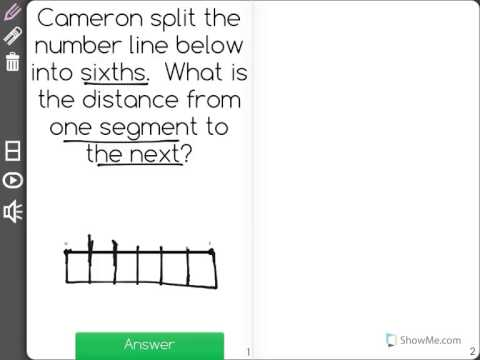By Freckle by Renaissance

Students must partition a number line into b equal parts, recognizing that each part has size 1/b. Front Row is a free, adaptive, Common Core aligned math program for teachers and students in kindergarten through eighth grade. Front Row allows students to practice math at their own pace - learning advanced concepts when they're ready and receiving remediation when they struggle. Front Row provides teachers with access to a detailed data dashboard and weekly email reports that show which standards are causing students difficulty, what small groups can be formed for interventions, and how their students are progressing in math.# [3.OA.3-1.0] Mult/Div within 100 - Common Core Standard

##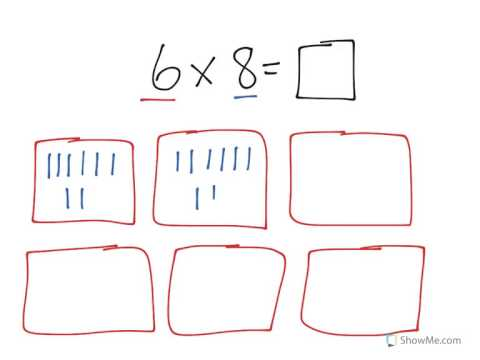By Front Row

Discover more Common Core Math at https://www.frontrowed.comUse multiplication within 100 to solve word problems in situations involving equal groups, arrays, and measurement quantities, e.g., by using drawings and equations with a symbol for the unknown number to represent the problem.1Front Row is a free, adaptive, Common Core aligned math program for teachers and students in kindergarten through eighth grade. Front Row allows students to practice math at their own pace - learning advanced concepts when they 're ready and receiving remediation when they struggle. Front Row provides teachers with access to a detailed data dashboard and weekly email reports that show which standards are causing students difficulty, what small groups can be formed for interventions, and how their students are progressing in math.Discover more Common Core Math at https://www.frontrowed.com# [3.OA.3-1.0] Mult/Div within 100 - Common Core Standard - Word Problem

##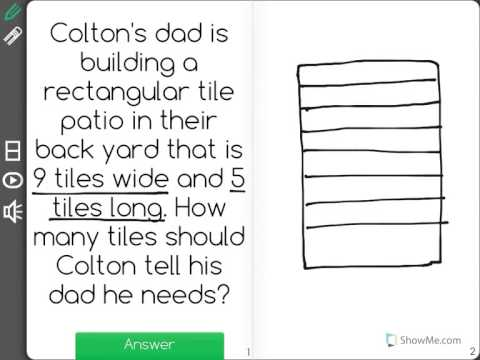By Front Row

Discover more Common Core Math at https://www.frontrowed.comUse multiplication within 100 to solve word problems in situations involving equal groups, arrays, and measurement quantities, e.g., by using drawings and equations with a symbol for the unknown number to represent the problem.1Front Row is a free, adaptive, Common Core aligned math program for teachers and students in kindergarten through eighth grade. Front Row allows students to practice math at their own pace - learning advanced concepts when they 're ready and receiving remediation when they struggle. Front Row provides teachers with access to a detailed data dashboard and weekly email reports that show which standards are causing students difficulty, what small groups can be formed for interventions, and how their students are progressing in math.Discover more Common Core Math at https://www.frontrowed.com# Math Basics: Division

##By GCFLearnFree.org

http://www.gcflearnfree.org/math Do you need help with basic math like subtraction multiplication division fractions times tables and percents? Our basic math tutorials and learning interactives make learning math easier and allow you to practice basic math skills at your own level and pace. Do you need to learn or practice the basic math skills of multiplication and division? How about some help with multiplication tables? The tips and techniques provided in this basic math tutorial make calculating multiplication and division simple easy and fun. What comes after multiplication in math? Division. Division is the opposite of multiplication. Instead of combining groups many times (like you do when you multiply) when you divide numbers you are splitting them into smaller equal groups. But you won't always have equal groups when you are dividing numbers or items -- sometimes you may have items left over. What do you do then? This lesson will help you figure that out by: • explaining the concept of dividing numbers • giving you division practice • helping you divide numbers that have remainders • showing you how to check your division If you are interested in learning more about this topic please visit our site to view the entire tutorial on our website. It includes instructional text informational graphics examples and even interactives for you to practice and apply what you've learned.# [5.NF.7c-1.0] Word Problems: Division - Common Core Standard

##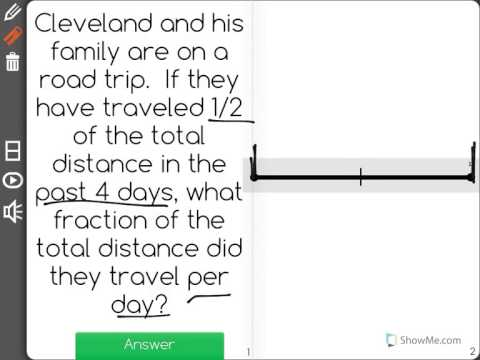By Freckle by Renaissance

Solve real world problems involving division of unit fractions by non-zero whole numbers and division of whole numbers by unit fractions, e.g., by using visual fraction models and equations to represent the problem. For example, how much chocolate will each person get if 3 people share 1/2 lb of chocolate equally? How many 1/3-cup servings are in 2 cups of raisins? Front Row is a free, adaptive, Common Core aligned math program for teachers and students in kindergarten through eighth grade. Front Row allows students to practice math at their own pace - learning advanced concepts when they're ready and receiving remediation when they struggle. Front Row provides teachers with access to a detailed data dashboard and weekly email reports that show which standards are causing students difficulty, what small groups can be formed for interventions, and how their students are progressing in math.# Multiplication as groups of objects

##By Khan Academy

Use arrays to show different ways to multiply and get the same solution.# Addition using groups of 10 and 100

##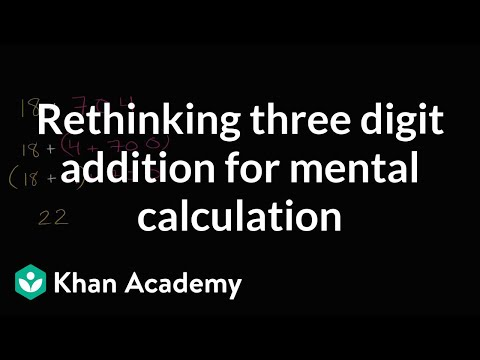By Khan Academy

Learn some techniques for doing mental addition, such as making groups of 10 and 100.# Addition using groups of 10 and 100

##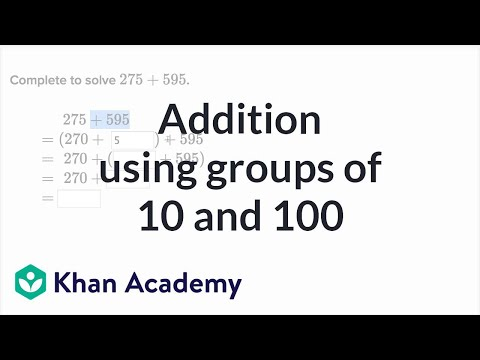By Khan Academy

Learn how to rewrite addition problems to make them easier to solve.# 3rd Grade Math Rap

##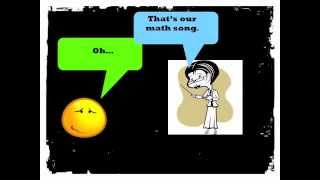By McCarthy Math Academy

With a little help from the group, Mindless Behavior, I have created a math video with lyrics to help my students to understand and apply core math skills for third grade. People of all ages can jam out to this one. Enjoy!

I've got a case of the operation blues.
Because I don't know which one I should use.
Look at the word problem for the clues.
The key words tell you how to choose.

Each means you multiply or you must divide.
Tryna find the total? Then you multiply
Total's in the problem? Then you must divide.
Not quick to solve it, draw it, get it right.

Addition's easy for me and you
Sum, In all, together, and total too.
When do you subtract? How many more?
Fewer? Left? Less? Difference in a score?

Place value's next. Disco on the " dess "
Ones, tens, hundreds, to the left
Thousands, Ten thousands, hundred...thousand
Say the name of the place, yeah.

The value's the amount of the place
For example, 2,060.
The value of the 2 is 2-0-0-0,
The value of the 6 is 6-0.

When you round, find and underline the place
Spotlight to the right, decide the digit's fate
5 or more, add 1 to the rounding place
4 or less, do nothing but walk away, (estimate)

A pen, penny is one, one cent
A Nic-kel is five, a dime is ten cents
25 for a quarter, George Washington
100 cents makes a dollar, there he goes again.

For pictographs, you gotta check out the key
One smiley face might really equal three
For bar graphs, pay attention to the scale
Think it's counting by ones, huh, you'll fail

Fractions are easy, just draw your best.
Here they go from least to greatest
1/12, 1/6, Â¼, 1/3,
Â½, 2/3, Â¾, Fraction nerd!

You see that number on top,
That's called the numerator
It describes the amount
That is being considered
And if you jump down from the fraction bar
Denominator
It's the total number of equal parts.

Let me give you an example:
Leslie Moin has some coins
A total of 9
2 happen to be pennies
While 7 are dimes.
What's the fraction of dimes?
How many coins? 9
How many dimes? 7
Say the fraction -- seven ninths

Length times width is Area
Distance around is Perimeter
Break down the GEOMETRY

3 sides makes triangle
4 sides = quadrilateral
5 pentagon, 6 hexagon
8 octagon, 10 decagon

Lines that never cross - PARALLEL
Lines that meet or cross - INTERSECTING
Lines that form right angles -- PERPENDICULAR

Same shape, same size -- CONGRUENT
Line that cuts in half - SYMMETRY
Up and Down - VERTICAL
Left to Right -- HORIZONTAL

An angle less than right - ACUTE
An angle opened wide - OBTUSE
Ninety degrees square corner - RIGHT ANGLE

Back to triangles
3 sides the same = equilateral
2 sides the same = isosceles
no sides the same = Hey, that's a scalene right!

So, that's it.
That's our math song.
Before we leave,
Remember to read
Your math problems three times before you answer.
That way you know what the problem
Is asking you to do.
Don't be lazy, be brilliant.
Piece! Like a fraction.# Place Value Song For Kids | Ones, Tens, & Hundreds | 1st - 3rd Grade

##By Lumos Learning

Explore our complete library of ad-free math videos at https://www.numberock.com​. Thank you for watching our Place Value Song. We hope you liked it!# Math Antics - Prime Factorization

##By mathantics# Math Antics - Fractions and Decimals

##By mathantics# Math Antics - What Is Arithmetic?

##By mathantics# Math Antics - Decimal Place Value

##By mathantics# Math Antics - Mixed Numbers

##By mathantics